Next: Eddy Currents Up: Magnetic Induction Previous: Magnetic Induction

## Motional Emf

We now understand how an emf is generated around a fixed circuit placed in a time-varying magnetic field. But, according to Faraday's law, an emf is also generated around a moving circuit placed in a magnetic field which does not vary in time. According to Eq. (201), no space-filling inductive electric field is generated in the latter case, since the magnetic field is steady. So, how do we account for the emf in the latter case?

In order to help answer this question, let us consider a simple circuit in which a conducting rod of length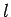slides along a U-shaped conducting frame in the presence of a uniform magnetic field. This circuit is illustrated in Fig. 36. Suppose, for the sake of simplicity, that the magnetic field is directed perpendicular to the plane of the circuit. To be more exact, the magnetic field is directed into the page in the figure. Suppose, further, that we move the rod to the right with the constant velocity.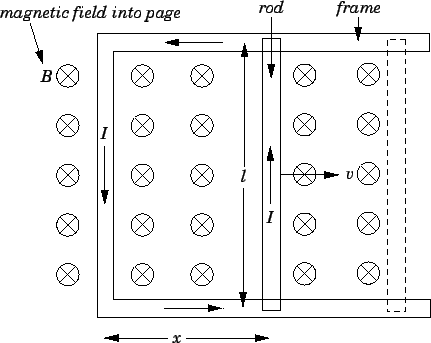The magnetic flux linked by the circuit is simply the product of the perpendicular magnetic field-strength,, and the area of the circuit,, wheredetermines the position of the sliding rod. Thus,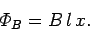(202)

Now, the rod moves a distancein a time interval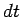, so in the same time interval the magnetic flux linking the circuit increases by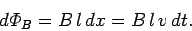(203)

It follows, from Faraday's law, that the magnitude of the emf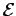generated around the circuit is given by(204)

Thus, the emf generated in the circuit by the moving rod is simply the product of the magnetic field-strength, the length of the rod, and the velocity of the rod. If the magnetic field is not perpendicular to the circuit, but instead subtends an anglewith respect to the normal direction to the plane of the circuit, then it is easily demonstrated that the motional emf generated in the circuit by the moving rod is(205)

whereis the component of the magnetic field which is perpendicular to the plane of the circuit.

Since the magnetic flux linking the circuit increases in time, the emf acts in the negative direction (i.e., in the opposite sense to the fingers of a right-hand, if the thumb points along the direction of the magnetic field). The emf,, therefore, acts in the anti-clockwise direction in the figure. Ifis the total resistance of the circuit, then this emf drives an anti-clockwise electric current of magnitudearound the circuit.

But, where does the emf come from? Let us again remind ourselves what an emf is. When we say that an emfacts around the circuit in the anti-clockwise direction, what we really mean is that a chargewhich circulates once around the circuit in the anti-clockwise direction acquires the energy. The only way in which the charge can acquire this energy is if something does work on it as it circulates. Let us assume that the charge circulates very slowly. The magnetic field exerts a negligibly small force on the charge when it is traversing the non-moving part of the circuit (since the charge is moving very slowly). However, when the charge is traversing the moving rod it experiences an upward (in the figure) magnetic force of magnitude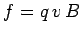(assuming that). The net work done on the charge by this force as it traverses the rod is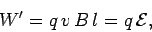(206)

since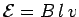. Thus, it would appear that the motional emf generated around the circuit can be accounted for in terms of the magnetic force exerted on charges traversing the moving rod.

But, if we think carefully, we can see that there is something seriously wrong with the above explanation. We seem to be saying that the charge acquires the energyfrom the magnetic field as it moves around the circuit once in the anti-clockwise direction. But, this is impossible, because a magnetic field cannot do work on an electric charge.

Let us look at the problem from the point of view of a chargetraversing the moving rod. In the frame of reference of the rod, the charge only moves very slowly, so the magnetic force on it is negligible. In fact, only an electric field can exert a significant force on a slowly moving charge. In order to account for the motional emf generated around the circuit, we need the charge to experience an upward force of magnitude. The only way in which this is possible is if the charge sees an upward pointing electric field of magnitude(207)

In other words, although there is no electric field in the laboratory frame, there is an electric field in the frame of reference of the moving rod, and it is this field which does the necessary amount of work on charges moving around the circuit to account for the existence of the motional emf,More generally, if a conductor moves in the laboratory frame with velocity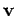in the presence of a magnetic field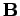then a chargeinside the conductor experiences a magnetic force. In the frame of the conductor, in which the charge is essentially stationary, the same force takes the form of an electric force, where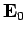is the electric field in the frame of reference of the conductor. Thus, if a conductor moves with velocitythrough a magnetic fieldthen the electric fieldwhich appears in the rest frame of the conductor is given by(208)

This electric field is the ultimate origin of the motional emfs which are generated whenever circuits move with respect to magnetic fields.

We can now appreciate that Faraday's law is due to a combination of two apparently distinct effects. The first is the space-filling electric field generated by a changing magnetic field. The second is the electric field generated inside a conductor when it moves through a magnetic field. In reality, these effects are two aspects of the same basic phenomenon, which explains why no real distinction is made between them in Faraday's law.Next: Eddy Currents Up: Magnetic Induction Previous: Magnetic Induction
Richard Fitzpatrick 2007-07-14